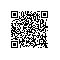# [python]Numpy快速入门

NumPY是Python的一个矩阵处理库，提供了大量点矩阵处理函数，其内部通过C语言实现，执行速度快。

#### 1.一维NumPY数组

>>> from NumPY import *
>>> a=arange(4)
>>> a
array([0, 1, 2, 3])
>>> a.shape
(4,)
>>> 

>>> mm=array((4,5,1))
>>> mm
array([4, 5, 1])
>>> pp=array((10,20,30))
>>> pp
array([10, 20, 30])
>>> mm+pp
array([14, 25, 31])
>>> pp*2
array([20, 40, 60])

>>> mm**2
array([16, 25,  1])

>>> mm
5
>>> pp
30
>>> 2*mm
10
>>> 

#### 2. 多维NumPY数组

>>> jj=array([[1,2,3],[4,5,6],[7,8,9]])
>>> jj
array([[1, 2, 3],
[4, 5, 6],
[7, 8, 9]])

>>> jj
array([4, 5, 6])
>>> jj
5
>>> jj[0,2]
3

>>> a1=array([1,2,3])
>>> b1=array([4,8,10])
>>> a1*b1
array([ 4, 16, 30])
>>> a2=array([[2,3,4],[5,6,7]])
>>> b2=array([[1,2,3],[4,5,6]])
>>> a2*b2
array([[ 2,  6, 12],
[20, 30, 42]])
>>> 

#### 3.NumPY矩阵

>>> from numpy import mat,matrix
>>> m1=mat([[1,2,3],[2,3,1]])
>>> m1
matrix([[1, 2, 3],
[2, 3, 1]])
>>> m2=mat([[1,1,2],[2,1,1]])
>>> m2
matrix([[1, 1, 2],
[2, 1, 1]])
>>> m1*m2
Traceback (most recent call last):
File "<stdin>", line 1, in <module>
File "/Library/Frameworks/Python.framework/Versions/3.5/lib/python3.5/site-packages/numpy/matrixlib/defmatrix.py", line 343, in __mul__
return N.dot(self, asmatrix(other))
ValueError: shapes (2,3) and (2,3) not aligned: 3 (dim 1) != 2 (dim 0)

>>> m2.T
matrix([[1, 2],
[1, 1],
[2, 1]])
>>> m1*m2.T
matrix([[9, 7],
[7, 8]])

>>> from numpy import multiply
>>> m1
matrix([[1, 2, 3],
[2, 3, 1]])
>>> m2
matrix([[1, 1, 2],
[2, 1, 1]])
>>> multiply(m1,m2)
matrix([[1, 2, 6],
[4, 3, 1]])

>>> m1.shape
(2, 3)

>>> m3=mat([[7,5,4],[9,8,7],[5,2,0]])
>>> m3
matrix([[7, 5, 4],
[9, 8, 7],
[5, 2, 0]])
>>> m3.sort()
>>> m3
matrix([[4, 5, 7],
[7, 8, 9],
[0, 2, 5]])

>>> m3.mean()
5.2222222222222223

>>> m3
matrix([[4, 5, 7],
[7, 8, 9],
[0, 2, 5]])
>>> m3[1,:]
matrix([[7, 8, 9]])
>>> m3[2,:]
matrix([[0, 2, 5]])

>>> m3[2,1:3]
matrix([[2, 5]])使用钉钉扫一扫加入圈子
+ 订阅